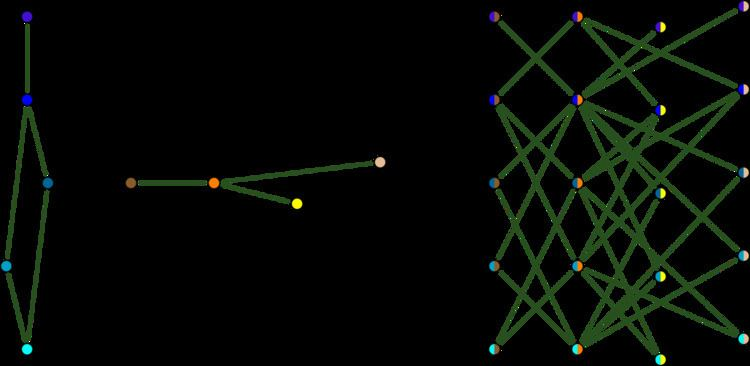# Tensor product of graphs

Updated onIn graph theory, the tensor product G × H of graphs G and H is a graph such that

## Contents

• the vertex set of G × H is the Cartesian product V(G) × V(H); and
• any two vertices (u,u') and (v,v') are adjacent in G × H if and only if
• u' is adjacent with v' and
• u is adjacent with v.
• The tensor product is also called the direct product, categorical product, cardinal product, relational product, Kronecker product, weak direct product, or conjunction. As an operation on binary relations, the tensor product was introduced by Alfred North Whitehead and Bertrand Russell in their Principia Mathematica (1912). It is also equivalent to the Kronecker product of the adjacency matrices of the graphs.

The notation G × H is also sometimes used to represent another construction known as the Cartesian product of graphs, but more commonly refers to the tensor product. The cross symbol shows visually the two edges resulting from the tensor product of two edges.

## Examples

• The tensor product G × K2 is a bipartite graph, called the bipartite double cover of G. The bipartite double cover of the Petersen graph is the Desargues graph: K2 × G(5,2) = G(10,3). The bipartite double cover of a complete graph Kn is a crown graph (a complete bipartite graph Kn,n minus a perfect matching).
• The tensor product of a complete graph with itself is the complement of a Rook's graph. Its vertices can be placed in an n by n grid, so that each vertex is adjacent to the vertices that are not in the same row or column of the grid.
• ## Properties

The tensor product is the category-theoretic product in the category of graphs and graph homomorphisms. That is, a homomorphism to G × H corresponds to a pair of homomorphisms to G and to H. In particular, a graph I admits a homomorphism into G × H if and only if it admits a homomorphism into G and into H.

To see that, in one direction, observe that a pair of homomorphisms fG : IG and fH : IH yields a homomorphism f: IG × H given by f(v) = (fG(v),fH(v)). In the other direction, a homomorphism f: IG × H can be composed with the projections homomorphisms πG : G × HG and πH : G × HH, given by πG((u,u' )) = u and πH((u,u' )) = u' , to yield homomorphisms to G and to H.

The adjacency matrix of G × H is the tensor product of the adjacency matrices of G and H.

If a graph can be represented as a tensor product, then there may be multiple different representations (tensor products do not satisfy unique factorization) but each representation has the same number of irreducible factors. Imrich (1998) gives a polynomial time algorithm for recognizing tensor product graphs and finding a factorization of any such graph.

If either G or H is bipartite, then so is their tensor product. G × H is connected if and only if both factors are connected and at least one factor is nonbipartite. In particular the bipartite double cover of G is connected if and only if G is connected and nonbipartite.

The Hedetniemi conjecture gives a formula for the chromatic number of a tensor product.

Topics